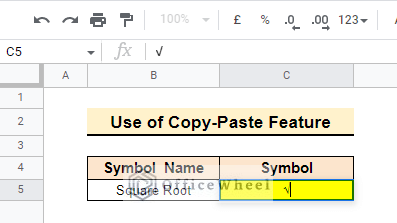# How to Insert Square Root Symbol (√) in Google Sheets (3 Ways)

You will have a hard time if you ever need to insert any special character or symbol in Google Sheets as there is no built-in method to do that. In terms of mathematical calculations, the square root symbol (√) is very useful. Don’t worry, here we will show you some easy approaches on how to insert the square root symbol in Google Sheets.

## 3 Easy Ways to Insert Square Root Symbol in Google Sheets

Assume you have a simple dataset that just contains 2 rows and 2 columns. The first column is for Symbol Names and the second column is for the corresponding Symbols. Here is a glimpse of the dataset. Suppose you need to insert the square root symbol in the symbol column.### 1. Apply Keyboard Shortcut to Insert Square Root Symbol

You can easily insert a square root symbol using a keyboard shortcut in Google Sheets. Just follow the step-by-step techniques below to do that.

📌 Steps:

• First, double-click on cell C5 and put the cursor where you want to insert the symbol.• Then, press Alt+251 from the numeric pad, release the Alt key, and press enter. In this way, you will get the square root symbol in the desired cell.Note: You must type 251 from the numeric pad otherwise the shortcut will not work. If there is no numeric pad in your laptop you can activate the num lock key and then apply the alt code.

### 2. Insert Square Root Symbol Using UNICHAR Function

There is another useful way in Google Sheets to insert the square root symbol. There is a built-in UNICHAR function in Google Sheets which works under specific ASCII codes. For the square root symbol, the code is 8730.

📌 Steps:

• First, apply the following formula in cell C5 to insert the square root symbol.
`=UNICHAR(8730)`• Now, if you want to insert the symbol with a number you have to modify the formula as shown below.
`=UNICHAR(8730)&4`• There is another way to insert the square symbol with a number and also show the output. To do that you have to modify the formula using the ampersand symbol (&) as follows.
`=UNICHAR(8730)&4&“=”&2`Note: There is no need to type the ASCII code from the numeric pad in the UNICHAR function.

Read More: How to Insert Division Symbol in Google Sheets (4 Quick Methods)

### 3. Use Copy-Paste Feature to Insert Square Root Symbol

Using the copy-paste feature is probably the most common way to insert any symbol or special character like the square root in Google Sheets. There are some different ways to do that as discussed below.

In this method, we will copy the square root symbol from Google Docs and then enter it in Google sheets.

📌 Steps:

• First, open a Google Doc on your computer. After that go to the Insert tab in the ribbon and click on the Special Characters options.• Then go to the Search Box in the popped-out window to search for the name of your desired symbol.• After that, type Square Root in the search box, and the symbol will show. Then, click on the square root symbol to insert it into the Google Doc file.• Now, the required symbol has been placed in your Google Doc file. Copy the symbol using CTRL+C.• Finally, open your Google Sheet and double-click the cell C5 where you want to insert the square root symbol and paste it using CTRL+V.#### 3.2 Using Windows Character Map

Copying special characters using Windows Character Map can be an effective alternative way. Follow the given steps to copy the Square Root symbol from Windows Character Map.

📌 Steps:

• First, enter “Character Map” in the search box on your computer. Then find and select the Character Map app from the list of results.• Then check the Advanced View checkbox.• Now, search for the square root symbol in the Search Box and then select it.• After that, make a copy of the selected symbol by pressing the Copy button.• Then, select the cell in Google Sheets where you want to insert the square root symbol and paste it using CTRL+V.

####As an alternative, you may just use your web browser to perform a Google search for the square root symbol. Then grab the symbol for the square root from the search results. The copied symbol should then be pasted into the applicable Google Sheets cell.

## How to Get the Square Root of a Number in Google Sheets

You can also use the SQRT function in Google Sheets to get the square root of numbers. For example, it will convert 16 to 4, 81 to 9, and so on. Follow the steps below to be able to do that.

📌 Steps:

• First, simply apply the formula given below in cell C5. Here cell B5 contains 100. So the formula returns 10 as it is the square root of 100.
`=SQRT(B5)`• Now, just drag the fill icon and you will get the square root value of the entire column.## Things to Remember

• You must type the ALT key code from the numeric pad. Otherwise, the shortcut may not work.
• Double-click on the symbol for selecting from the character map.

## Conclusion